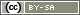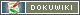# loopToPage()

`int loopToPage(string pageID, int repititions)`

The function `loopToPage()` is used to show the pages of the current page with the ID pageID multiple times, as often as the number of repititions are set.

This function specifies how often the pages have been repeated. In the first round = 0, second round = 1 etc.

Important: The function `loopToPage()` can not be used simultaneously with `loopPage()`, `setNextPage()` or `setPageOrder()`.

• pageID ID of the last page from the series of pages to be repeated (pageID).
• repititions The number of times the series of pages is to be repeated.

Advice: Use the function `loopIndex()` to see on current pages of the series how often it has been repeated until then.

## 1st example

You want to repeat page 5 to 8 of the survey 3 times with different questions each time.

• change the ID of page 8 to “loopend” (you can choose the ID as you wish)
• place the PHP-Code (Introduction to PHP) on page 5
`loopToPage('loopend', 3);`

Now you want to have question `A001` to `A005` on the first round. On the second round you want question `A101` to `A105` to show up on the page. And on the third round question `A201` to `A205`

(the questions were created in section `A0` and the section has been copied twice by downloading it as a file and importing it again).

On page 5 you place the PHP-Code shown in the picture. It produces an array which contains a list of the questions and makes them available for the following pages via `registerVariable()`

```\$fragen = array(
0 => array('A001', 'A002', 'A003', 'A004', 'A005'),
1 => array('A101', 'A102', 'A103', 'A104', 'A105'),
2 => array('A201', 'A202', 'A203', 'A204', 'A205')
);
registerVariable(\$fragen);```

To show the first two questions on the first page of the loop (page 5) you have to add the `loopPage()` code as the picture demonstrates:

```\$i = loopToPage('loopend', 3);
// now retrieve the part relevant for the current pass from \$fragen
\$set = \$fragen[\$i];
// show the first two questions from the set
question(\$set);
question(\$set);```

To show the next questions on page 6 you have to place the following PHP-code on the page. The command `loopPage()` shows in which round the page is revealed. The remaining code is equivalent to the code shown above.

```\$i = loopIndex();
\$set = \$fragen[\$i];
// show the next question (index 2)
question(\$set);```

Repeat the code from above for page 7 and 8. Change the number `2` from the last line to `3` and `4`.

## 2nd example

Like in the first example you want to repeat the pages 5 to 8. You gave page 8 the ID “loopend”. Earlier on page 3 you asked a question with 10 possible free mentions as answers (`ON01`). You want to repeat the following pages for every answer given.

You copied the section `A0` from the catalogue of questions nine times (section `A1` to `A9`). Accordingly the questions on page 5 are defined as follows:

```\$fragen = array(
0 => array('A001', 'A002', 'A003', 'A004', 'A005'),
1 => array('A101', 'A102', 'A103', 'A104', 'A105'),
2 => array('A201', 'A202', 'A203', 'A204', 'A205'),
3 => array('A301', 'A302', 'A303', 'A304', 'A305'),
4 => array('A401', 'A402', 'A403', 'A404', 'A405'),
5 => array('A501', 'A502', 'A503', 'A504', 'A505'),
6 => array('A601', 'A602', 'A603', 'A604', 'A605'),
7 => array('A701', 'A702', 'A703', 'A704', 'A705'),
8 => array('A801', 'A802', 'A803', 'A804', 'A805'),
9 => array('A901', 'A902', 'A903', 'A904', 'A905')
);
registerVariable(\$fragen);```

To make it more elegant you can also use a FOR-loop. Alternatively you can use the following PHP-code:

```\$fragen = array();
// compile all ten repititions (index 0 to 9)
for (\$i=0; \$i<=9; \$i++) {
\$rubrik = 'A'.\$i;  // A0 to A9
\$fragen[\$i] = array();
// list question 01 to 05 in the current part
for (\$f=1; \$f<=5; \$f++) {
\$fragen[\$i][\$f-1] = \$rubrik.sprintf('%02d', \$f);
}
}```

Now it has to be specified how many “free mentions” the participant answered. With the command `registerVariable()` all answers are made available for the following pages. Along with that the length of the list is determined with the command `count()`. The length of the list matches the loops of the pages. The command `loopToPage()` initializes the loops.

```\$items = getItems('ON01', 'valid');
registerVariable(\$items);
\$i = loopToPage('loopend', count(\$items));```

Now you have to determine the correct set of questions with `\$items` and the number of repititions. From this list you can e.g. show the first two questions on page 5.

```\$id = \$items[\$i];  // \$i was determined by loopToPage() (see above)
\$set = \$fragen[\$id];
// see 1st example
question(\$set);
question(\$set);```

And now the complete PHP-Code for page 5:

```\$fragen = arrray(
0 => array('A001', 'A002', 'A003', 'A004', 'A005'),
1 => array('A101', 'A102', 'A103', 'A104', 'A105'),
2 => array('A201', 'A202', 'A203', 'A204', 'A205'),
3 => array('A301', 'A302', 'A303', 'A304', 'A305'),
4 => array('A401', 'A402', 'A403', 'A404', 'A405'),
5 => array('A501', 'A502', 'A503', 'A504', 'A505'),
6 => array('A601', 'A602', 'A603', 'A604', 'A605'),
7 => array('A701', 'A702', 'A703', 'A704', 'A705'),
8 => array('A801', 'A802', 'A803', 'A804', 'A805'),
9 => array('A901', 'A902', 'A903', 'A904', 'A905')
);
registerVariable(\$fragen);

\$items = getItems('ON01', 'valid');
registerVariable(\$items);
\$i = loopToPage('loopend', count(\$items));

\$id = \$items[\$i];
\$set = \$fragen[\$id];
question(\$set);
question(\$set);```

On the pages 5 to 8 just the bottom part is used with slight modifications:

```\$i = loopIndex();
\$id = \$items[\$i];
\$set = \$fragen[\$id];
question(\$set);  // index 2 shows question 3, because the index counting starts with 0```